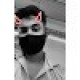In the given figure product of the elements in the row (b*d) is same as the product of the elements in the column (a*b*c). There are 6 possible numbers to fill in the figure 2, 7 , 11, 14 , 3 , 21.

Which of the above numbers has to be in the list

1. 2
2. 3
3. 7
4. 11

See our previous Puzzle of the day

Puzzle of the day: 159

1.ansh sethi

7

2.Gaurang Sahu

option 3 i.e. 7

because our main motive is to make
a*c = d
therefore, d can be
d=14 or d=21
in which 7 is common hence,
option 3.

3.7

4.it’s 7

5.7. (2,7,14) (3,7,21)

• In the island of Hoola Boola Moola , inhabitants have a...

By Sanket211, 4 days ago

• For a=0.9086, x= 1.0286 and a= -1.6627, x= 4.9173

By Aditya Dang, 1 month ago

• Since, x+1 satisfies the equation ax^2+ bx+ c=0. Theref...

By Aditya Dang, 1 month ago

• The HCF of 141 and 517 is 47. But 47 does not completel...

By Aditya Dang, 1 month ago

• 1. How many integral pairs (x, y), where 0< x, y <...

By lastknight99, 1 month ago

• Hi, It is given that a= (212)b . Forming an equation...

By Aditya Dang, 1 month ago

• Perimeter of circle is 2pi(793)^1/2.

By Aditya Dang, 1 month ago

• Area of ΔDOC is 21cm^2.

By Aditya Dang, 1 month ago

• Length of smaller of two sides is equal to 36 units.

By Aditya Dang, 1 month ago

• Length of AB is (17)^1/2 units.

By Aditya Dang, 1 month ago

• The sum borrowed is Rs. 3300.

By Aditya Dang, 1 month ago

• Two natural numbers a and b are given in base 10. The n...

By kanzzz, 1 month ago